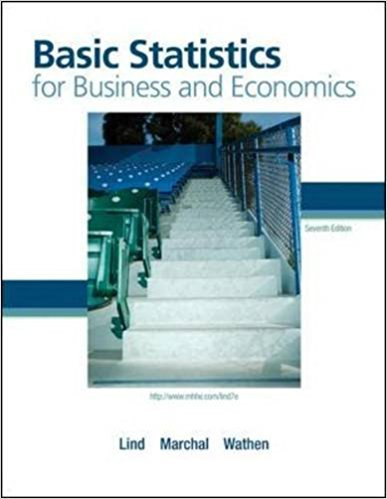×
Get Full Access to Basic Statistics For Business And Economics - 7 Edition - Chapter 4 - Problem 11e
Get Full Access to Basic Statistics For Business And Economics - 7 Edition - Chapter 4 - Problem 11e

×

# The following values are the starting salaries, in \$000,ISBN: 9780077384470 64

## Solution for problem 11E Chapter 4

Basic Statistics for Business and Economics | 7th Edition

• Textbook Solutions
• 2901 Step-by-step solutions solved by professors and subject experts
• Get 24/7 help from StudySoup virtual teaching assistantsBasic Statistics for Business and Economics | 7th Edition

4 5 1 323 Reviews
20
5
Problem 11E

Problem 11E

The following values are the starting salaries, in \$000, for a sample of five accounting graduates who accepted positions in public accounting last year.

 36 26 33 28 31

a. Determine the mean, median, and the standard deviation.

b. Determine the coefficient of skewness using Pearson’s method.

c. Determine the coefficient of skewness using the software method.

Step-by-Step Solution:

Solution

Step 1 of 3

a) We have to find mean, median and standard deviation for the given data

The given data is 36, 26, 33, 28, 31

Arrange the values in the ascending order 26, 28, 31, 33, 36

Here n=no.of value=5

Mean= ( 26+28+31+33+36)/5

=30.8

Median===31

Standard deviation====3.96

Hence Mean=30.8

Median=31

standard deviation =3.96

Step 2 of 3

Step 3 of 3

##### ISBN: 9780077384470

This textbook survival guide was created for the textbook: Basic Statistics for Business and Economics , edition: 7. This full solution covers the following key subjects: determine, Accounting, coefficient, Using, skewness. This expansive textbook survival guide covers 6 chapters, and 325 solutions. The full step-by-step solution to problem: 11E from chapter: 4 was answered by , our top Business solution expert on 08/23/17, 08:36AM. Since the solution to 11E from 4 chapter was answered, more than 2338 students have viewed the full step-by-step answer. Basic Statistics for Business and Economics was written by and is associated to the ISBN: 9780077384470. The answer to “The following values are the starting salaries, in \$000, for a sample of five accounting graduates who accepted positions in public accounting last year.36.026.033.028.031.0a. Determine the mean, median, and the standard deviation.________________b. Determine the coefficient of skewness using Pearson’s method.________________c. Determine the coefficient of skewness using the software method.” is broken down into a number of easy to follow steps, and 49 words.

Unlock Textbook Solution